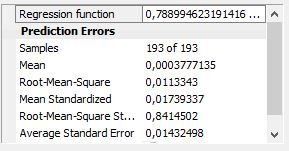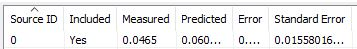# understanding cross validation errors

87
6
09-15-2020 02:11 AM

Hello ArcGIS community,

I'm trying to understand how different errors of cross validation are calculated. I've the formula of desktop.arcgis.com page, but I could not find out for some of them. I'm using the ozone tutorial data. I've attached 2 files. Thank you to answer to these questions :

1- How is calculated the standard error for an individual sample ? from wich data ? I've tried unsuccessfuly different combinaison

2- How is calculated the average standard error ? I've found a different value for the average of the individual standard errors.

But not for the stadardized error and the mean standardized, where I could recalculate standardized error from the standard error and the mean standardized from the mean of the individual standard errors.

3- How is calculated the RMS error ? is that from the whole prediction data or only from the cross validation data ?

4- which standard error we use for standardizing the RMS ?

Is it possible, if you have time, to make a summary of how calculate all these parameters, it can be usefull for the whole community, as understanding the prediction errors is as important than prediction itself.

Thank you so much for taking time to answer.

Tags (1)
6 Replies
byEsri Regular Contributor

Hello,

Below are the exact formulas for the cross validation statistics, taken from page 279 this pdf:

https://dusk.geo.orst.edu/gis/geostat_analyst.pdf

When cross-validating a point, the remaining points produce a cross-validation prediction and standard error.  All cross-validation statistics and summary statistics are based on these two numbers, along with the measured value at the point.

Keep in mind that the cross-validated prediction and standard error of a point are not the same as the final interpolated prediction and standard error at that point.  The former is calculated by removing the point, and the latter is calculated by including it.  This is likely why your calculations don't match the numbers in the Geostatistical Wizard.

Trying to reproduce the cross-validation results on your own is not simple.  If you would like, I can explain how to do it for a single point.

-Eric

Note: There is actually a typo in the Average kriging standard error formula below.  The sigma-hat inside the sum should be squared.  Though it's called "Average kriging standard error," it would probably be more technically correct to call it the "Root mean cross validation variance."Thank you very much for the quick response.

Yes if you can explain to me with a small example the procedure for calculating these different parameters, because this will be the only way for me to understand, not being familiar with mathematical formulas, and please specifying for each case if the data are those of the final interpolation or of the cross validation:

1- the standard error for an individual sample
2- the average standard error
3- the RMS error
4- the standard error we use for standardizing the RMS

about the forgotten square in the 2009 formula, I have a corrected version. but a small difference in the title of the formula brought me a little clarification: in my version the word kriging has been removed from "average kriging standard error". what would be the exact expression?

thanks again.

byEsri Regular Contributor

To reproduce the cross-validation statistics for a single point:

1. Create a Kriging geostatistical layer in the Geostatistical Wizard using all points (you probably have already done this).
2. On the points that were used to create geostatistical layer, deselect the point that you want to cross-validate.  In other words, every other point in the dataset should be selected.
3. Use the Create Geostatsitical Layer geoprocessing tool.  Provide the geostatistical layer and the points with the selection into the tool.  The output will be a new geostatistical layer that used the kriging parameters from the first layer and applied them to the dataset with the selection.
4. Invert the selection so that only the point you want to cross-validate is selected.
5. Use the GA Layer To Points geoprocessing tool.  Use the geostatistical layer created in step 3, and predict to the single selected point.  Provide the field containing the measured value.
6. The output will contain all of the cross-validation statistics for the single feature.

To calculate the summary statistics like RMSE, you'll need to do this for every feature in the dataset, then plug the values into the formulas in that PDF.

I highly suggest that you don't try to do this manually.  The Cross Validation geoprocessing tool was created exactly for this purpose.

Dear Erik,
I thank you deeply for your answers which I am sure are very clear. However, this is not exactly what could be of use to me. I do not intend to calculate the parameters by myself. But, until I did it for a little example, I couldn't figure it out.

So if I am still allowed to abuse your time, I pose my problem in another way. But I'm sure your answer will help a lot of people in the community.

From my experience in teaching, it is often the calculation of uncertainties that poses the most problems of understanding, and finally we find ourselves confronted during research work to see very nice models, but whose reliability and validity remains to be proven for lack of objective analysis of estimation errors.

then, if you can explain us through the example of the pdf that you sent (page 54) which explains very well the principle of calculation of the kriging until the final estimate for an unsampled point by finally giving its variance and its standard error (page 58). until then I understood perfectly, well I think.

Question 1- is it this standard error which is used to calculate the "average standard error" in "capture3.jpg"? is it the same in "Capture4.JPG"? if yes then it's ok.Let's now take 1 point and give it an estimate by cross-validation: let's say sample (1,3) without doing the calculation let's say its new value is "104", with the error is 105-104=1.

Question 2: Could you calculate its standard error like in "Capture5.JPG"?Question 3- How is calculated the RMS error "Capture3.JPG"? is that from the whole prediction data or only from the cross validation data?

Question 4- which standard error we use for standardizing the RMS "Capture3.JPG"?

sorry to bother you and thank you again ..

Cheers.

byEsri Regular Contributor

Whenever kriging "predicts" at a new location, it calculates both a kriging prediction and a kriging variance (the standard error is the square root of the variance).  The formulas for these values are complicated, but they are based on the semivariogram and the locations/values of neighboring points.  You can see these formulas in the pdf, but they are not simple to explain and require understanding of linear algebra and Lagrange multipliers.

When a point is being cross-validated, its value is hidden, and the kriging prediction and kriging variance at the location of the hidden point is calculated based on every other point.  We call these the cross-validated prediction and cross-validated variance of the point.  The "Predicted" field of the table represents the cross-validated prediction, and the "Standard Error" field represents the square root of the cross-validated variance. The "Measured" field is the true value of the hidden point, and the "Error" field is the difference between the Predicted and Measured.

Once these statistics are calculated for every point by sequentially hiding each of them, the summary statistics like Average Standard Error can be calculated.  The Mean Error, for example, is the simple average of the Error column.  The Average Standard Error is the square root of the average of the cross-validated kriging variances.  To calculate it by hand, square all of the values of the Standard Error field, take the average of the squared values, and take the square root of the result.  You can see the formulas for all of these summary statistics in my first post.

In the formulas, z(s) refers to the measured value at a location.  z^(s) refers to the cross-validated prediction (the "hat" ^ appears above z(s)). σ-hat refers to the cross-validated standard error (the square root of the variance).

The actual computation of these numbers is less important than what they're measuring.  The RMSE and Average Standard Error are important because they both estimate uncertainty of predicted values in different ways, and if the uncertainty is being estimated consistently, both numbers should be approximately equal.  If they differ significantly, this is a sign that uncertainties are not being modeled effectively.  Similarly, the standardized RMS directly measures whether standard errors are being under- or over-estimated.

It doesn't answer to my questions. But it's fair enough. Thank you anyway.

Is there any french version of the ArcGIS pdfs ?

Anybody can provide the exact french translation of :

- Root Mean Square Error

- Root Mean Square Standardized Error

- Average Standard Error

Regards